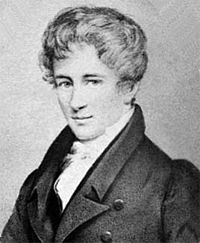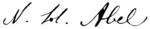# Niels Henrik Abel facts for kids

Kids Encyclopedia Facts
Niels Henrik AbelNiels Henrik Abel
Born 5 August 1802
Nedstrand, Denmark-Norway
Died 6 April 1829 (aged 26)
Froland, Norway
Nationality Norwegian
Alma mater Royal Frederick University (BA, 1822)
Known for Abel's binomial theorem
Abelian category
Abelian variety
Abel equation
Abel equation of the first kind
Abelian extension
Abel function
Abelian group
Abel's identity
Abel's inequality
Abel's irreducibility theorem
Abel–Jacobi map
Abel–Plana formula
Abel–Ruffini theorem
Abelian means
Abel's summation formula
Abelian and tauberian theorems
Abel's test
Abel's theorem
Abel transform
Abel transformation
Abelian variety
Abelian variety of CM-type
Dual abelian variety
Scientific career
Fields Mathematics